Next: Worked example 10.1: Equilibrium Up: Statics Previous: Ladders and walls

## Jointed rods

Suppose that three identical uniform rods of mass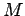and length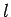are joined together to form an equilateral triangle, and are then suspended from a cable, as shown in Fig. 94. What is the tension in the cable, and what are the reactions at the joints?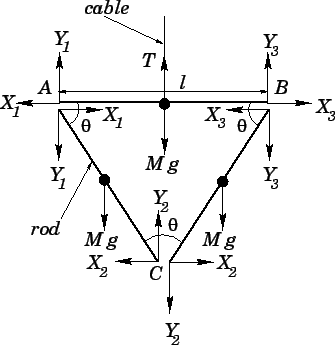Let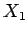,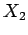, and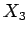be the horizontal reactions at the three joints, and let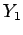,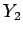, and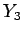be the corresponding vertical reactions, as shown in Fig. 94. In drawing this diagram, we have made use of the fact that the rods exert equal and opposite reactions on one another, in accordance with Newton's third law. Let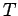be the tension in the cable.

Setting the horizontal and vertical forces acting on rod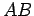to zero, we obtain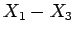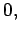(491)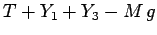(492)

respectively. Setting the horizontal and vertical forces acting on rod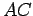to zero, we obtain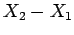(493)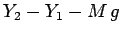(494)

respectively. Finally, setting the horizontal and vertical forces acting on rod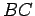to zero, we obtain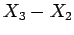(495)(496)

respectively. Incidentally, it is clear, from symmetry, that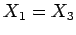and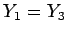. Thus, the above equations can be solved to give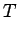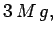(497)(498)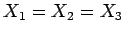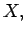(499)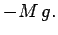(500)

There now remains only one unknown,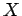.

Now, it is clear, from symmetry, that there is zero net torque acting on rod. Let us evaluate the torque acting on rodabout point. (By symmetry, this is the same as the torque acting on rodabout point). The two forces which contribute to this torque are the weight,, and the reaction. (Recall that the reactionis zero). The lever arms associated with these two torques (which act in the same direction) are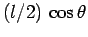and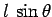, respectively. Thus, setting the net torque to zero, we obtain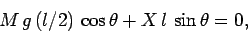(501)

which yields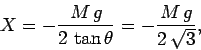(502)

since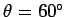, and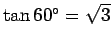. We have now fully determined the tension in the cable, and all the reactions at the joints.Next: Worked example 10.1: Equilibrium Up: Statics Previous: Ladders and walls
Richard Fitzpatrick 2006-02-02